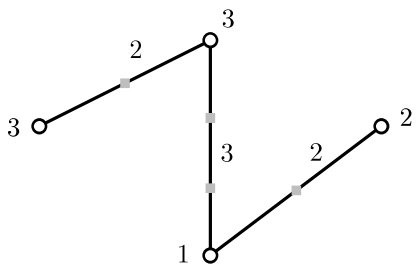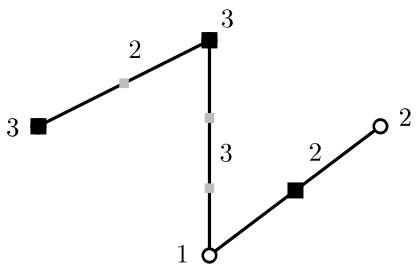시간 제한메모리 제한제출정답맞은 사람정답 비율
4 초 1024 MB0000.000%

## 문제

ICP(International Carrier Products) Company plans to build new $k$ logistical warehouses in order to facilitate the efficient delivery of products. Before being delivered to the target location, the products are stored at a logistical warehouse. Then these products are delivered to the final location for distribution. The locations of logistical warehouses are very important in optimizing delivery time and space of warehouse logistics.

Imagine that the chain for supply and management is given as a tree $T = (V, E)$. Each node $v_i$ of $T$ has weight $w_i$, and each edge $e_j$ of $T$ has an integer length $l_j$. For a point $p$ in an edge, the distance from a node $v_i$ to $p$ is defined to be $w_i \times |\pi(v_i, p)|$, where $\pi(v_i, p)$ is the path connecting $v_i$ and $p$ in $T$ and $|\pi(v_i, p)|$ is the sum of the lengths of segments (edges) in $\pi(v_i, p)$.

We want to choose $k$ points on the edges of $T$ as centers under the following restriction: each center lying on an edge $e$ must be a point at an integer distance from each endpoint of $e$. Observe that centers are allowed to lie on nodes. For instance, if the length of $e$ is $3$, we can choose a center among the following four points: the two endpoints of $e$ and the two points on $e$ at distance $1$ from the endpoints of $e$.

The goal is to choose $k$ centers on the edges of $T$ such that the maximum distance from a node to its closest center is minimized. We call a set of such $k$ centers an optimal set of $k$ centers for this problem.

For example, figure (a) shows a tree with four nodes of weights $3$, $3$, $1$, $2$ labelled next to the vertices, and three edges with lengths $2$, $3$, $2$ labelled next to the edges. Centers are allowed to lie on the nodes and the small gray squares. If we choose three centers among them, an optimal solution is shown in the black squares of figure (b) with the maximum distance $2$.(a) (b)

Given a tree $T = (V, E)$ of $n$ nodes with weights and $n - 1$ edges with integer lengths, write a program to output the maximum distance from a node to its closest center in an optimal set of $k$ centers.

## 입력

Your program is to read from standard input. The input starts with a line containing two integers $n$ and $k$ ($1 \le k \le n \le 200,000$), where $n$ is the number of nodes in a tree and $k$ is the number of centers to choose. Then there are $n-1$ edges in the tree. The nodes are numbered from $1$ to $n$, and the edges are numbered from $1$ to $n-1$. The next line contains $n$ positive integers such that the $i$-th integer represents the weight of the $i$-th node. The weights are no more than $10^6$. In the following $n-1$ lines, the $i$-th line contains three positive integers. The first two integers represent the two indices of the nodes at the endpoints of the $i$-th edge. The third integer represents the length of the $i$-th edge. The lengths are no more than $10^6$.

## 출력

Your program is to write to standard output. Print exactly one line. The line should contain the maximum distance from a node to its closest center in an optimal set of $k$ centers.

## 예제 입력 1

2 1
2 3
1 2 3


## 예제 출력 1

4


## 예제 입력 2

3 2
12 2 1
1 2 1
2 3 3


## 예제 출력 2

2


## 예제 입력 3

4 3
3 3 1 2
1 2 2
2 3 3
3 4 2


## 예제 출력 3

2


## 예제 입력 4

6 2
7 4 3 4 12 3
6 5 4
2 3 3
5 1 12
4 5 3
1 2 4


## 예제 출력 4

15